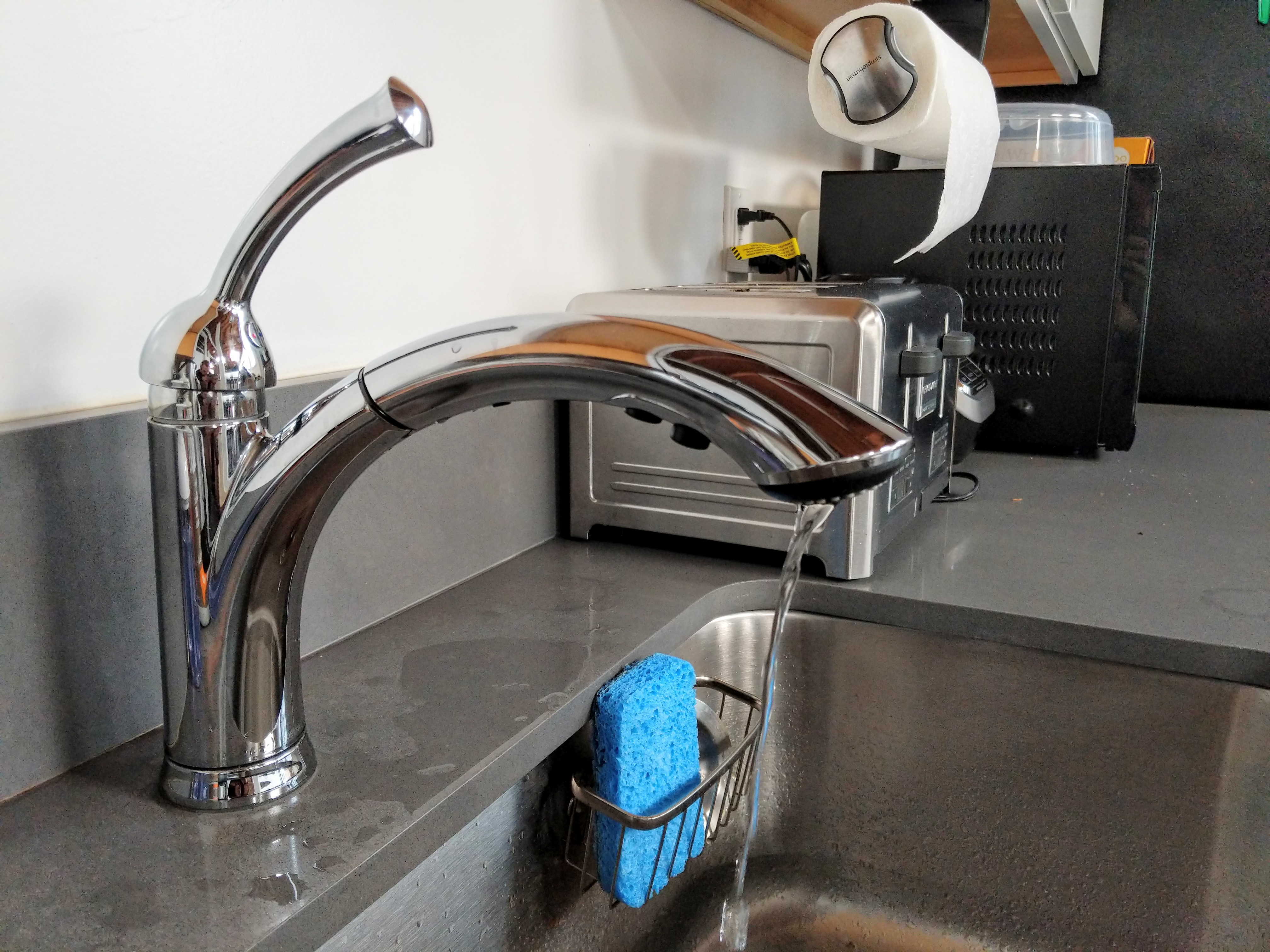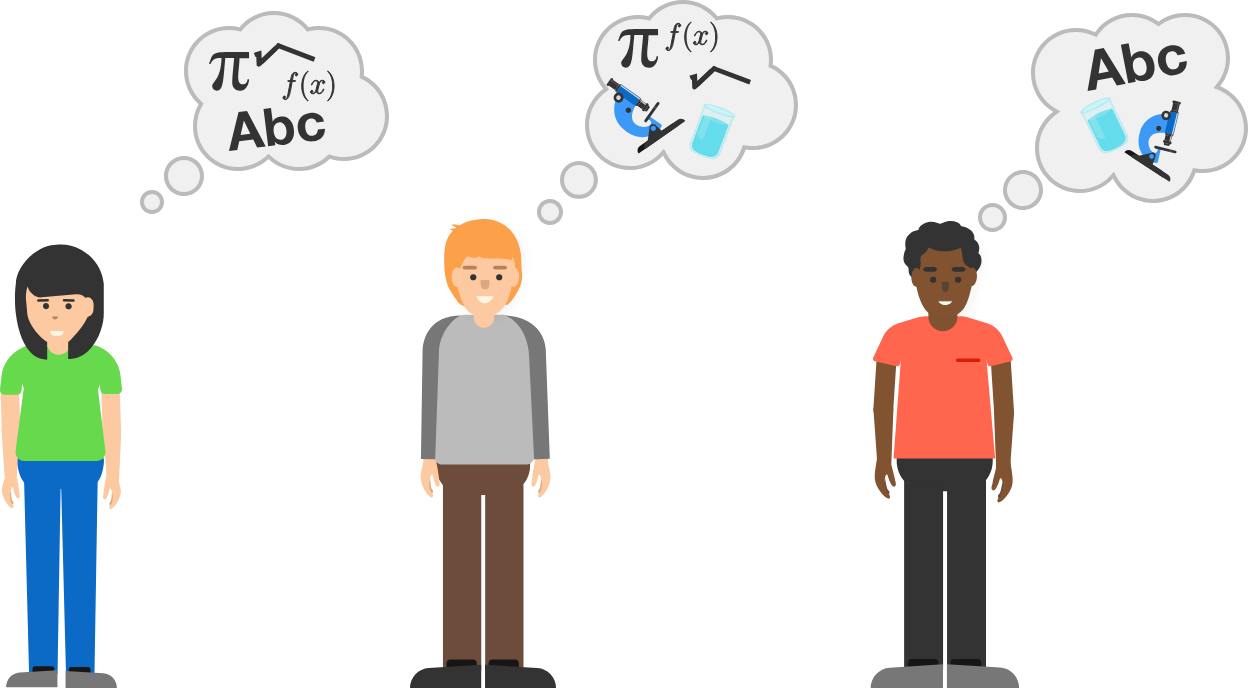# Problems of the Week

Contribute a problem

# 2018-11-05 BasicOnce fully turned on, an old kitchen faucet produces a slow but steady flow of water, free of bubbles and turbulent flow.

Thanks to the pull of gravity, the stream of water accelerates as it falls.

Does the shape of the stream change as the water falls?

In a particular school, English, math, and science are taught to all students, where

• some students who like English also like math;
• some students who like math also like science;
• some students who like science also like English.

Is there at least 1 student in the school who likes English, math, and science?$A$ and $B$ are two distinct positive numbers such that their average is equal to $C.$

Is it possible for the average of $A^2$ and $B^2$ to be $C^2?$

If $f(x)=x^2,$ then $f\big(f(x)\big)=x^4.$

Are there any other functions $f(x)$ that satisfy $f\big(f(x)\big)=x^4?$

Suppose you have $X$ marbles and I have $Y$ marbles, for a total of 2020.

• Firstly, you give me half of the marbles that you have.
• Then, I give you half of the marbles that I have at that moment, including the marbles that you have just given me.

Amazingly, I am left with the same number of marbles as one of us originally had before the exchanges!

How many marbles do you have now?

×

Problem Loading...

Note Loading...

Set Loading...